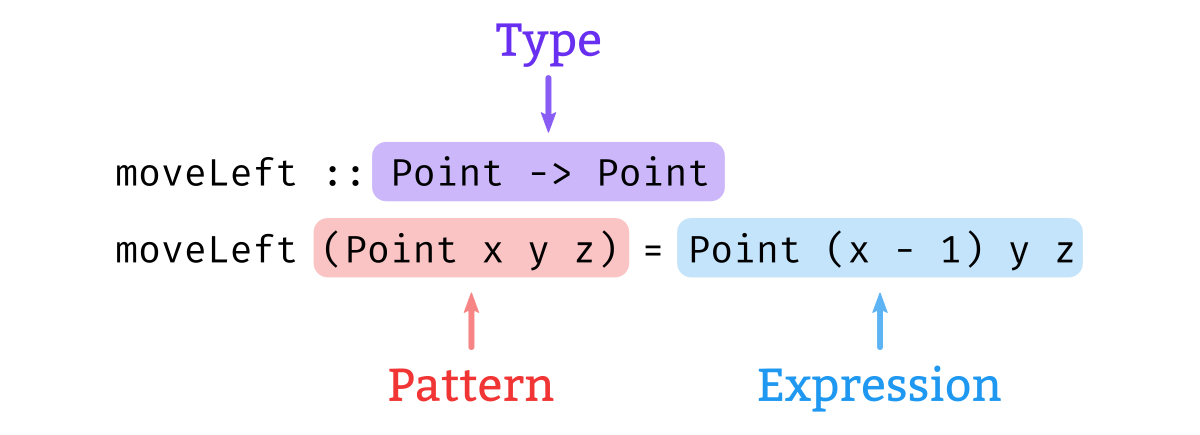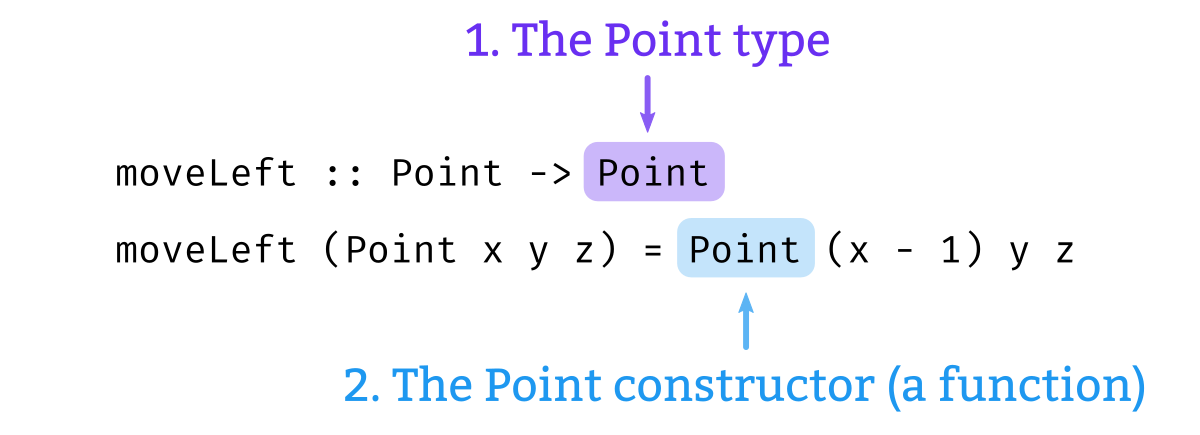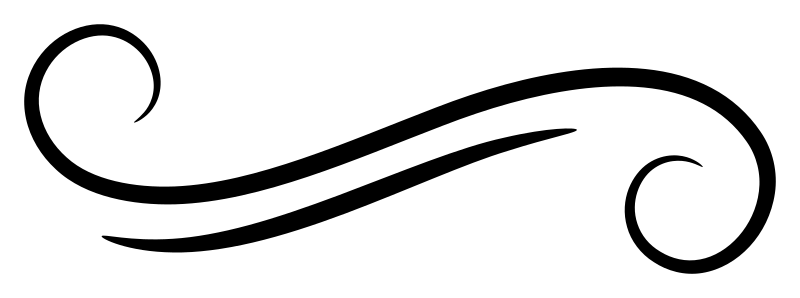# Data classes

Python classes are “a means of bundling data and functionality together.” Data classes are a recent addition to PythonData classes first appeared in Python 3.7, released in June 2018. that place a heavier emphasis on the data than the functionality aspect. This invites a pleasant comparison with Haskell datatypes, which exhibit a more distinct separation between data and functionality.

The following example defines a type representing a point in three dimensions as the conjunction of three integers:

``````from dataclasses import dataclass

@dataclass
class Point:
x: int
y: int
z: int``````

This class looks and behaves quite similarly to the following Haskell datatype defined using record syntax:

``````data Point =
Point
{ x :: Integer
, y :: Integer
, z :: Integer
}
deriving (Eq, Show)``````

Data classes can also have all the complexities of regular Python classes, and the `dataclass` decorator is a good deal more feature-packed than we discuss here. We will focus on the primary purpose of data classes: the field list and the special methods that are generated based on the field list.

## Init

`__init__` is a special methodSpecial methods are sometimes called “dunder” methods (for the double underscores in their names). that initializes the instance; it is called when a value is first constructed. In the example above, the `dataclass` decorator in the example above generates the following method for the `Point` class:

``````def __init__(self, x: int, y: int, z: int) -> None:
self.x = x
self.y = y
self.z = z``````

The effect is roughly akin to what the constructor of a Haskell record does. Here’s how we create a new `Point` value, in Python and in Haskell:

``````>>> Point(3,4,5)
Point(x=3, y=4, z=5)``````
``````λ> Point 3 4 5
Point {x = 3, y = 4, z = 5}``````

## Types vs constructors

In both languages, one may find it slightly unsettling that the word “Point” describes two things:

1. The type;
2. A function that returns a value of that type.

How can “Point” be overloaded to mean both of those things? The answer varies between the two languages. The Python and Haskell REPL sessions above look more or less identical, but if you’re interested in the subtle distinctions about what’s going on “under the hood”, as they say, read on.

The Python expression `Point`, by itself, refers to the class. We can see this by typing `Point` into the REPL:

``````>>> Point
<class '__main__.Point'>``````

The reason we can write the expression `Point(3,4,5)` is that Python classes are callable; this means they have the special method `__call__`. The Python documentation describes how the expression desugars:

if this method is defined, `x(arg1, arg2, ...)` is a shorthand for `x.__call__(arg1, arg2, ...)`.

We can verify in the REPL that this method is in fact defined on `Point`:

``````>>> Point.__call__
<method-wrapper '__call__' of type object at 0x20cc148>``````

And we can also evaluate the desugared form and see that it does produce the same result.

``````>>> Point.__call__(3,4,5)
Point(x=3, y=4, z=5)``````

In Haskell, the distinction between `Point` the type and `Point` the function is made by context. We will use the following function definition to illustrate.This function accepts one `Point` argument, and returns a new `Point` where the `x` coordinate is reduced by one. Interpreted according to the convention that the axis labeled X in three-dimensional space is oriented with lesser numbers to the left and greater numbers to the right, this function’s output is one unit to the left of its input, and so we have chosen to name it `moveLeft`.

``````moveLeft :: Point -> Point
moveLeft (Point x y z) = Point (x - 1) y z``````

“Point” appears multiple times here: In a type context, in a pattern context, and in an expression context.• What comes after two colons (`::`) is a type.
• What comes before the equal symbol (`=`) is a pattern. As a pattern, a constructor splits up a value into its consituent parts.
• What comes after the equal symbol (`=`) is an expression. As an expression, a constructor builds a value from its constituent parts.

From the contexts in which they appear, then, we can tell:1. “Point” refers to the `Point` type
2. “Point” is a function that constructs a value of the `Point` type.

## Deriving

The other special methods that data classes generate automatically correspond to Haskell typeclass deriving.

• `__repr__` – equivalent to deriving `Show`
• `__eq__` – equivalent to deriving `Eq`.
• `__lt__`, `__le__`, `__gt__`, and `__ge__` If the `dataclass` function is called with `order=True`. – equivalent to deriving `Ord`.
• `__hash__` If the `dataclass` function is called with `frozen=True`. – equivalent to deriving `Hashable`, which we discuss below.

## Replacing field values

The `replace` function lets you construct a new instance based on an existing one, with one or more field values modified.

``````>>> from dataclasses import replace

>>> p = Point(3,4,5)

>>> p
Point(x=3, y=4, z=5)

>>> replace(p, x=0)
Point(x=0, y=4, z=5)``````

Somewhat uncharacteristically, this is not accomplished with a function in Haskell, but instead is a feature of the built-in record syntax.

``````λ> p = Point 3 4 5

λ> p
Point {x = 3, y = 4, z = 5}

λ> p { x = 0 }
Point {x = 0, y = 4, z = 5}``````

## Hashing

Hashing is important in Python because it is the basis of the `set` and `dict` structures. For some variety we’ll switch up the example; this time we’ll define a data class called `Color`:

``````@dataclass
class Color:
red: int
green: int
blue: int
opacity: float``````

Suppose we want to make a `set` of the primary colors.

``````>>> set([ Color(255,0,0,1), Color(0,255,0,1), Color(0,0,255,1) ])
TypeError: unhashable type: 'Color'``````

`Color`s can’t go into a set unless they’re hashable. We can get that by requesting a frozen data class, which entreats Python to generate an implementation of the `__hash__` function.

``````@dataclass(frozen=True)
class Color:
red: int
green: int
blue: int
opacity: float``````

Now we have a hash function for `Color`:

``````>>> green = Color(81, 143, 81, 1)

>>> hash(Color(255,0,0,1))
7323420538949561947

>>> hash(replace(green, opacity = 0.5))
4851574255485823697``````

And thus now we can put `Color`s into a set.

``````>>> set([ Color(255,0,0,1), Color(0,255,0,1), Color(0,0,255,1) ])
{Color(red=0, green=255, blue=0, opacity=1), Color(red=0, green=0, blue=255, opacity=1), Color(red=255, green=0, blue=0, opacity=1)}``````

Haskell doesn’t have hashing as a built-in concept. Instead of hash-based collections, we more often use the `Ord`-based collectionsSee the containers package on Hackage. found in the `containers` library.

But we do have hash-based collections`HashSet` is defined in the `unordered-collections` package. in Haskell as well. If we want to store a collection of colors in a `HashSet`, then the `Color` type must have an instance of the `Hashable` typeclass. We will need to turn to the `hashable` librarySee the hashable package on Hackage. and a few language extensions.For more about what’s going on here, see our page on deriving strategies.

``````{-# LANGUAGE DeriveAnyClass,
DerivingStrategies, DeriveGeneric #-}

import GHC.Generics (Generic)
import Data.Hashable

data Color =
Color
{ red :: Integer
, green :: Integer
, blue :: Integer
, opacity :: Double
}
deriving stock (Show, Generic)
deriving anyclass (Hashable)``````
``````λ> hash (Color 255 0 0 1)
7967393144227192017

λ> hash (Color 0 255 0 1)
204075674505530803``````

## Default values

Python’s data classes can have default values for its fields, just like Python functions can have defaults for their parameters. Haskell does not have a concept of parameter defaults, so this is something we have to reckon with.

Let’s look at the first example from PEP 557:

``````@dataclass
class InventoryItem:
'''Class for keeping track of an item in inventory.'''
name: str
unit_price: float
quantity_on_hand: int = 0``````

The default value of `0` for `quantity_on_hand` in the Python data class allows us to construct an `InventoryItem` with only two parameters instead of three:

``````>>> InventoryItem("Banana", 10)
InventoryItem(name='Banana', unit_price=10, quantity_on_hand=0)``````

Our corresponding Haskell type declaration has no ability to specify default values for its fields.Note that this example uses floating-point numbers to represent money, which is not generally a good idea due to the possibility of rounding error. Consider using the safe-money package if you deal with money.

``````-- | An item in inventory.
data InventoryItem =
InventoryItem
{ name :: String
, unitPrice :: Double
, quantityOnHand :: Integer
}
deriving (Eq, Show)``````

As an analogue in Haskell, we might write a function with two parameters.

``````-- | An inventory item that is out of stock.
inventoryItem
:: String  -- ^ Name
-> Double  -- ^ Unit price
-> InventoryItem
inventoryItem name unitPrice =
InventoryItem
{ name = name
, unitPrice = unitPrice
, quantityOnHand = 0
}``````

Or more tersely using record wildcards:

``````{-# LANGUAGE RecordWildCards #-}

inventoryItem
:: String  -- ^ Name
-> Double  -- ^ Unit price
-> InventoryItem
inventoryItem name unitPrice =
InventoryItem{quantityOnHand = 0, ..}``````

Now we can use the `inventoryItem` function like we used the Python `InventoryItem` constructor:

``````λ> inventoryItem "Banana" 10
InventoryItem {name = "Banana", unitPrice = 10.0, quantityOnHand = 0}``````

## Instance methods

There is one more piece of the example from PEP 557 that we haven’t mentioned yet: the `total_cost` method on the `InventoryItem` class.

``````@dataclass
class InventoryItem:
'''Class for keeping track of an item in inventory.'''
name: str
unit_price: float
quantity_on_hand: int = 0

def total_cost(self) -> float:
return self.unit_price * self.quantity_on_hand``````

We do not typically attach functions to data in this way in Haskell. The `total_cost` function becomes a top-level function in the Haskell version.We use the `fromIntegral` function to convert from `Integer` to `Double` (or, more generally, from any integer-like type to any other type of number).

``````totalCost :: InventoryItem -> Double
totalCost x =
unitPrice x * fromIntegral (quantityOnHand x)``````

Alternatively, using the `RecordWildCards` extension:

``````totalCost :: InventoryItem -> Double
totalCost InventoryItem{..} =
unitPrice * fromIntegral quantityOnHand``````Join Type Classes for courses and projects to get you started and make you an expert in FP with Haskell.